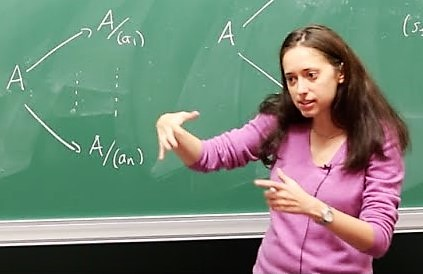Topos theory and Caramello's bridge technique Peter Arndt Department of Mathematics University of Düsseldorf (Germany)   This tutorial will offer an introduction to topos theory and geometric logic, and to the theory of topos-theoretic bridges developed by O.Caramello [1,2]. Grothendieck toposes can be seen as common generalization of the concepts of a universe of sets and of a topological space. There is an abundance of examples from topology, algebraic geometry, differential geometry and logic. A Grothendieck topos is a kind of category, in which one can interpret the language of geometric logic, a certain infinitary first order language, in a way that generalizes the usual set-theoretic interpretation. Geometric logic is an intuitionistic infinitary first order logic based on that language, which is sound and complete with respect to the topos interpretation. As usual, for the completeness part one has to show that if a theory 𝕋 does not imply a formula φ, then there is a model of 𝕋 in some topos where φ is not satisfied. In topos theory, the completeness theorem takes a particularly nice form: there exists a topos B[𝕋], and a model of 𝕋 in it which satisfies only those sentences implied by 𝕋, and thus takes care of all sentences φ as above simultaneously. The topos B[𝕋] is called the classifying topos of the theory 𝕋, and the said model of 𝕋 is called the universal model. Every model of 𝕋 in some topos arises as an image of the universal model. Like a group can be presented by generators and relations between them, a Grothendieck topos can be presented by a site, i.e. a small category together with a specification of when a family of morphisms with common codomain is a covering of that codomain. The inspiring example is the category of open subsets of a topological space together with the usual notion of covering from topology. Just as different presentations can give rise to isomorphic groups, different sites can give rise to equivalent toposes.Given a geometric theory, one can explicitly construct a site presenting its classifying topos, the syntactic site of T. It can happen that two different theories, giving rise to two di erent sites, have equivalent classifying toposes. Caramello's bridge technique studies and exploits such situations: one can try to translate properties of the classifying topos into properties of the theories, and back, and thus obtain relations between the two di erent theories. In this tutorial we will introduce Grothendieck toposes, the interpretation of geometric logic in them, classifying toposes and Caramello's bridge technique, all with examples. The prerequisite for the course is knowledge of the basic notions of category theory: categories, functors, natural transformations, (co)limits, adjunctions and the Yoneda lemma. Bibliography O.Caramello, Theories, Sites, Toposes: Relating and studying mathematical theories through topos-theoretic `bridges', Oxford University Press, 2017. O.Caramello, Grothendieck toposes as unifying `bridges' in Mathematics, habilitation thesis, University of Paris 7, 2016. S.MacLane & I.Moerdijk, Sheaves in Geometry and Logic, Springer, 1994. M.Makkai & G.Reyes, "First-Order Categorical Logic", Lecture Notes in Mathematics, vol. 611, Springer, 1977. Back to the 6th Universal Logic School !